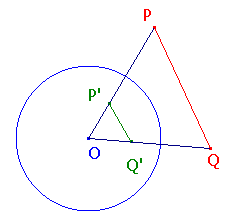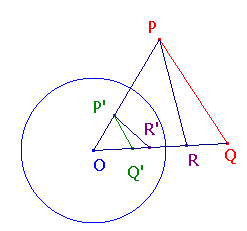Proof of The Equal Angles Theorem and its Corollary

The Equal Angles Theorem

Suppose that C is a circle with centre O, and that P and Q are points other than O.
Let P and Q have inverses P' and Q' with respect to C.
Then <QPO = <OQ'P' (in magnitude and sense)

Proof
Let r denote the radius of C.
As P and P' are inverse, OP.OP' = r2.
As Q and Q' are inverse, OQ.OQ' = r2.
Thus, OP.OP' = OQ.OQ', so OP/OQ = OQ'/OP'.
Hence, triangles OPQ and OQ'P' are similar. (Note the order of the vertices!)
It follows that the given angles are equal in magnitude and sense.Corollary to the Equal Angles Theorem

Suppose that P, Q and C are as in the theorem, and that R lies on the line OQ.
Let R' be the inverse of R with respect to C.
Then <QPR = -<Q'P'R' (in magnitude and sense)

Proof